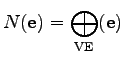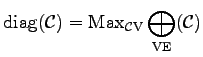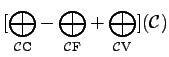### Number of Neighboring Edges of a Vertex

In order to estimate the number of diagonals of a multi-diagonal system matrix for instance for an equation system, it has to be determined how many vertices are connected to a vertex via an edge. As generally assumed, an edge is bounded by exactly two vertices. The number of incident edges and the number of vertices which are connected via an edge to a given vertex is identical. For this reason it is only necessary to count the edges which are incident with a vertex. The formula expressing this fact can be written as follows:(2.53)

In order to estimate the number of matrix diagonals, the maximum of neighboring edges within the cell complex has to be determined.(2.54)

In some applications it is necessary to obtain global information of the underlying cell complex. This mostly depends on the fact whether such a complex features a hole or consists of two non-connected subregions. These cases have to be discriminated before a sensible mathematical consideration is possible. The Euler number  is determined in the following manner from the number of cells, edges and vertices of a cell complex. This can be easily achieved by the following operation:(2.55)

One main advantage compared to the standard formulation is that this is an explicit and unambiguous definition, which is directly executable by a computer.

Michael 2008-01-16